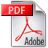Back to 3/2018
General and Professional Education
3/2018 pp. 17-23

## The possibilities of experimental verification of quantum theories of metals at the level of technical studiesGet full text pdf

Abstract

The authors present the advantages and disadvantages of introducing quantum elements of metal physics into the curriculum of engineering studies. On the one hand, the rapid development of new technologies forces future engineers to acquaint with the foundations of quantum theory of metals, but on the other hand, these theories use complex mathematical formalism, which requires additional hours in the physics program. What is more, not all quantum theories lead to correct results. This paper presents the foundations of two quantum theories that lead directly to analytical equations: free electron theory and Hartree-Fock theory. Then, using the theoretical equations, we calculate the bulk modulus of the metals and compare the obtained results with experiment. Then we analyze the causes of discrepancies occurring in both theories.

Key words

quantum elements of metal physics, free electron theory, Hartree-Fock theory

References

1. Auletta, G., Foundations and Interpretation of Quantum Mechanics. World Scientific, London, 2000.

2. Treagust, D.F., Chittleborough, G., Mamiala, T.L., Students’ understanding of the role of scientific models in learning science, Int. J. Sci. Educ., 24, 357, 2002.

3. Greca, I.M., Moreira, M.A., Mental, physical, and mathematical models in the teaching and learning of physics, Sci. Educ., 86, 106, 2002.

4. Gilbert, J.K., Models and modelling: routes to more authentic science education, Int. J. Sci. Math. Educ. 2, 2004.

5. Hadamard, J., Psychologia odkryć matematycznych, PWN, Warszawa, 1964.

6. Shaposhnikova, T.O., Hadamard, J.,: A Universal Mathematician, American Mathematical Soc., 1999.

7. Ashcroft, N.W., Mermin, N.D., Solid State Physics, Harcourt, Orlando, 1976.

8. Kittel, Ch., Introduction to Solid State Physics, Wiley, New York, 2004.